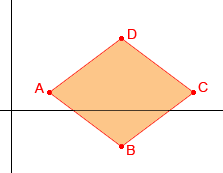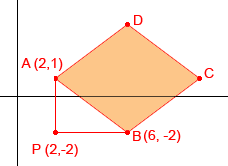SEARCH HOMEMath Central Quandaries & QueriesSubject: rhombus Name: jose Who are you: Student show mathematically that a quadrilateral whose vertices are A(2,1),B(6,-2) C(10,1),and D(6,4) is a rhombusHi Jose,

I would first construct a quick sketch to make sure it looks like a rhombus.It does look like a rhombus so now I have to decide how I will show that the four sides have equal lengths. I could use the distance formula (which is just Pythagoras' Theorem in an algebraic form) but I would rather use Pythagoras' Theorem directly to find the length of each side. For example to find the length of AB add a point P(2,-2) which forms a right triangle APB.The length of AP is 1 + 2 = 3 and the length of PB is 6 - 2 = 4 and hence from Pythagoras' Theorem the length of BA is

Sqrt(32 + 42) = Sqrt(9 + 16) = Sqrt(25) = 5

Now find the lengths of the remaining 3 sides.

PennyMath Central is supported by the University of Regina and The Pacific Institute for the Mathematical Sciences.